# 1st PUC Physics Model Question Paper 2 with Answers

Students can Download 1st PUC Physics Model Question Paper 2 with Answers, Karnataka 1st PUC Physics Model Question Papers with Answers helps you to revise the complete Karnataka State Board Syllabus and score more marks in your examinations.

## Karnataka 1st PUC Physics Model Question Paper 2 with Answers

Time: 3.15 Hours
Max Marks: 70

General Instructions:

1. All parts are compulsory.
2. Draw relevant figure / diagram wherever necessary.
3. Numerical problems should be solved with relevant formulae.

Part – A

I. Answer the following questions : ( 10 × 1 = 10 )

Question 1.
Define velocity.
The rate of change of differ cement is known as velocity.

Question 2.
What is a null vector?
A vector whose magnitude is zero and that has any arbitrary direction is known as a null or zero vector.

Question 3.
W hat is the unit of measurement of energy used in our electricity bills?
kWh

Question 4.
Where is the centre of mass of a meter stick?
at 50 cmQuestion 5.
Name the natural satellite of earth.
Moon.

Question 6.
What is hydraulic stress?
Hydraulic stress is the normal compressible force acting per unit area of the immersed body.

Question 7.
Why is the tip of the nib of a pen split?
The tip of the nib of a pen is split in order to provide a capillary which helps the ink to rise to the end of the nib and enables it to write continuously.

Question 8.
Why some cooking pots have copper coating on the bottom?
Copper is an excellent conductor of heat. The copper coating on the bottom of pots enables the cooking process quicker.

Question 9.
State the law of equipartition of energy.
For gases in thermal equilibrium, the energy supplied to the gas is equally distributed among the degrees of freedom and molecules, so that energy per molecule per degree of freedom is $$\left(\frac{1}{2}\right)$$ KBT where kB is Boltzmann’s constant.Question 10.
Define amplitude of a wave.
The maximum displacement of the wave is known as its amplitude.

Part – B

II. Answer any FIVE of the following questions: ( 5 × 2 = 10 )

Question 11.
Name a fundamental force which has long range.and a fundamental force which has short range of operation.

• Short range – strong nuclear force.
• Long range – Gravitational force.

Question 12.
Distinguish between path length and displacement.
Distance covered:

• It is the change in position of a particle without the mention of a direction.
• It is the actual length of the path covered by the particle.

Displacement:

• It is change in position of a particle in a specified direction.
• It is the shortest distance between the initial and the final point described by the particle.Question 13.
The position of a particle is given by $$r=3.0 t \hat{i}+2.0 t^{2} \hat{j}+5.0 \hat{k}$$ where t is in seconds and the coefficients have the proper units for r to be in metres. Find v(t) of the particle.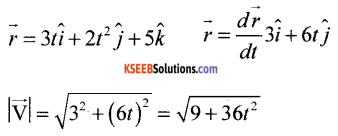Question 14.
What is impulse and impulsive force?
A huge force acting on a body for a short interval of time is known as an impulsive force. Impulse is defined as the product of mass of the body and change in velocity of the body. Impulse = m(v – u).

Question 15.
State and explain Boyle’s law.
At constant temperature, the volume of a given mass of a gas is inversely proportional to its pressure.
V ∝ $$\frac{1}{\mathrm{P}}$$ at constant temperature
So that P1V1 = P2V2.Question 16.
What is reversible process? Give an example.
A process is reversible if it can be reversed such that both the system and the surrounding return to their original states.

Question 17.
A planet has same mass as that of earth, but its radius is half that of earth. If the acceleration due to gravity on earth is ‘g’, what is the acceleration due to gravity on that planet.
Acceleration due to gravity on earth.
$$g_{E}=\frac{\mathrm{GM}}{\mathrm{R}^{2}}$$
If mass (M) of the planet is equal to the mass of the earth and radius (R) is half $$\left(\frac{\mathrm{R}}{2}\right)$$ of the earth, the accleration due to gravity on that planet.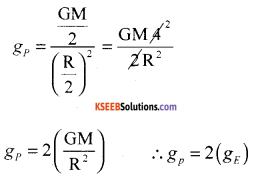∴The acceleration due to gravity on that planet is twice the acceleration due to gravity on the earth.Question 18.
Mention the expression for kinetic energy of a particle executing simple harmonic motion. Explain the terms.
$$\mathrm{K.E}=\frac{1}{2} m \omega^{2} \mathrm{A}^{2} \sin ^{2}(\omega t+\phi)$$
$$\mathrm{K.E}=\frac{1}{2} \mathrm{k} \mathrm{A}^{2} \sin ^{2}(\omega t+\phi)$$
Where A → Amplitude
m → mass of the particles
W → angular frequency
t → time
k → force constant

Part – C

III. Answer any FIVE of the following questions : ( 5 × 3 = 15 )

Question 19.
Consider an equation v = v0+ at, where v0 is the initial velocity, v is the final velocity, a is the acceleration and t is the time taken. Check whether this equation is dimensionally correct.
Ans. v = v + at
[LT-1 ] = [LT-1 ] + [LT-2 ][T1 ]
(LT-1) = [LT-1] + [LT-1]
Since energy term on the RHS has the same dimensional formula, hence the equation is dimensionally correct.

Question 20.
Derive an expression for centripetal acceleration.
Let $$\vec{r}$$ and $$\vec{r}^{\prime}$$ be the position vectors and $$\vec{v}$$ and $$\vec{v}^{\prime}$$ veIocities of the object when it is at point P and P’. By definition, velocity at a point is along the tangent at that point in the direction of the motion. Since the path is circular $$\vec{v}$$ is perpendicular to $$\vec{r}$$ and $$\vec{v}^{\prime}$$ is perpendicular to $$\vec{r}^{\prime}$$.
Therefore, ∆$$\vec{v}$$is perpendicular to ∆$$\vec{r}$$ Average acceleration $$\frac{\Delta \vec{v}}{\Delta t}$$ is perpendicular to ∆$$\vec{r}$$.
The magnitude of $$\vec{a}$$ is, by definition, given by $$|\vec{a}|=\lim _{\Delta t \rightarrow 0} \frac{|\Delta \vec{v}|}{\Delta t}$$
The triangle formed by the position vectors is similary to the triangle formed by the velocity vectors.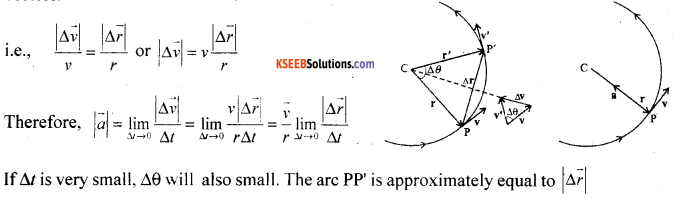lf ∆t is very small. ∆ will also small. The arc PP is approximately equal to $$|\Delta \vec{r}|$$
i.e., $$\lim _{\Delta t \rightarrow 0} \frac{|\Delta \vec{r}|}{\Delta t}$$ = v. Thus, centripetal acceleration $$|\vec{a}|=\frac{v}{r} v=\frac{v^{2}}{r}$$ and $$\vec{a}=\frac{v}{r} \frac{d \vec{r}}{d t}$$.
The centripetal acceleration is always directed towards the centre. The centripetal force = ma.Question 21.
Write any one disadvantage of friction. What are the different methods of reducing friction?

1. Friction hinders motion of bodies.
2. Friction causes wear and tear of machinery parts.
3. Wear and tear can be minimised by polishing the surfaces and lubricating machinery parts with oil and grease etc.
4. Streamlining the bodies reduce fluid (air or water) resistance.

Question 22.
Differentiate between conservative force and non-conservative force with one example for each.
The force is said to be conservative, if the work done by the force in moving a particle over a closed path is zero.
Eg: Electrostatic force .
The force is said to be non-conservative, if the work done by the force over a closed path is not zero.
Eg: viscous force is a liquid.

Question 23.
Find the force required to stretch a wire of area of cross section 2 x 10-4 m2 so that its length becomes 1.5 times original length. Young’s modulus = 3.6 x 1011 Nm-2.
Y = $$\frac{\text { stress }}{\text { strain }}$$
A = 2 × 10-4 m2
∆l = 1.51
Y = 3.6 × 1011 Nm-2
F= ?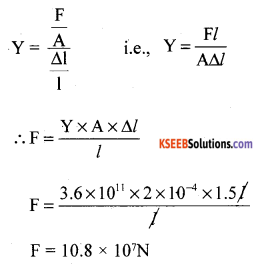Question 24.
State Pascal’s law. Give two applications of Pascal’s law.
The total normal force exerted by a fluid on any surface in contact with it, is called fluid thrust. Devices which use the principles of pascal’s law are,

• Hydraulic brakes and
• Sphygmo manometer (used to measure blood pressure).

Question 25.
Determine the molar specific heat at constant volume for a mono atomic gas molecule.
(dQ) = du = Cv dT
ie. Cv dT = $$\frac{f \mathrm{R} d \mathrm{T}}{2}$$
∴ Cv = $$\frac{f R}{2}$$ for mono atonic gas, f = 3
Cv = $$\frac{3 \mathrm{R}}{2}$$

Question 26.
Give the theory of beats.
Let y1 = A sin ω1t and y2 = A sin ω2t represent two progressive waves travelling in the same direction and superpose with each other.
y = y1 + y2 = A ( sin ω1t + sin ω2t )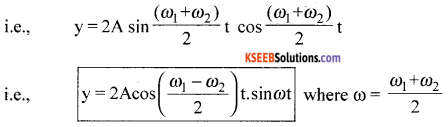and ωb = ω1 – ω2 or fb = f1 – f2 where fb is known as the beat frequency and tb = $$\frac{1}{f_{b}}=\frac{1}{f_{1}-f_{2}}$$ as the beat period.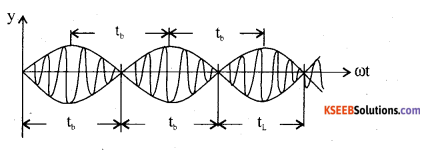Part – D

IV. Answer any TWO of the following questions : ( 2 × 5 = 10 )

Question 27.
What is a projectile? Derive the equation of path of a projectile.
A particle thrown into the space and whose motion is guided by the action of gravity is called as a projectile.
Let v be the velocity of projection. Let θ be the angle of projection. Let v0x = v0 cosθ and v0y = v0 sinθ represent X and Y components of velocities.
For the motion in (x, y) plane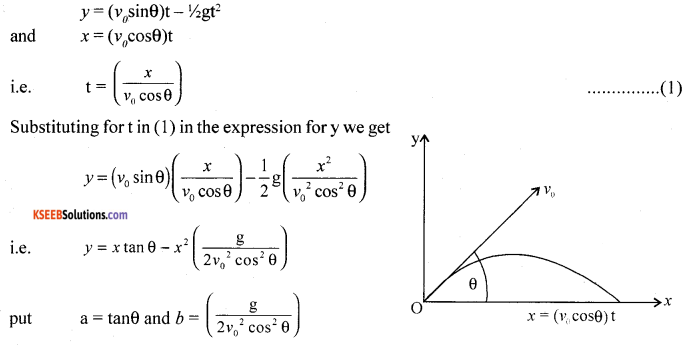We get y = ax – bx2 which is quadratic equation representing equation of a parabola.
Hence trajectory of a projectile describes a parabola.Question 28.
Illustrate the law of conservation of mechanical energy in case of a ball dropped from a cliff of height H.
Energy can neither be created nor destroyed but can be transformed into other forms of energy.
Let a particle be at ‘C’. Initially the particle w ill be zero velocity. Let ‘h’ be the height of the particle.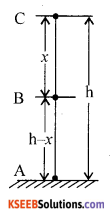P.Ec = mgh, K.Ec = 0
T.E = mgh
At B, K.E = $$1 / 2 \mathrm{mv}_{\mathrm{B}}=1 / 2 \mathrm{m}(2 \mathrm{g} x)=\mathrm{mg} x$$
P.EB = mg (h – x)
Hence T.E = (mgh – mgx) + (mgx)
= mgh
At A, vA2= 2gh
So that K.E = $$\frac{1}{2}$$ m2gh = mgh
P.EA = O.
Hence at all points A, B and C, the total energy is conserved.

Question 29.
Define moment of inertia of a body. State and explain perpendicular axis theorem and parallel axis theorem of moment of inertia.
Statement : The moment of inertia about an axis perpendicular to two other axes acting in the same plane with their point of intersection being a point on it and the (third) axis passing through the common point, is equal to the sum of moments of inertia about the two axes.
e.g: Iz = Ix + Iy.
Let M be the mass of the disk of radius R.
M.I. about a point passing through the centre and perpendicular to the plane containing X and Y is I = $$\frac{M R^{2}}{2}$$
Since X and Y are in the same plane, Ix = Iy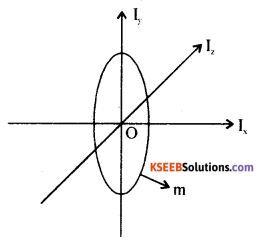∴ Iz = Ix + Iy becomes Iz = 2Ix
hence Ix = $$\frac{I_{Z}}{2}=\frac{M R^{2}}{4}$$
i.e. moment of inertia of a circular disc about the diameter $$\frac{I_{Z}}{2}=\frac{M R^{2}}{4}$$

V. Answer any TWO of the following questions : ( 2 × 5 = 10 )

Question 30.
Derive the expression for acceleration due to gravity at a point below the surface of earth. What is the value of acceleration due to gravity at the centre of the earth?
Let ‘p’ be the mean density of the Earth. Let ‘R’ be the radius of the Earth.
Mass of the Earth ME = Vρ
ME = $$=\frac{4}{3} \pi R_{E}^{3} \rho$$
Hence acceleration due to gravity,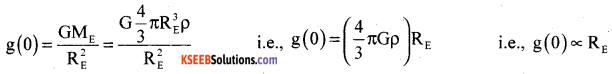Acceleration due to gravity at a depth ‘d’ due to radius REand mass ME, g(d) ∝ RE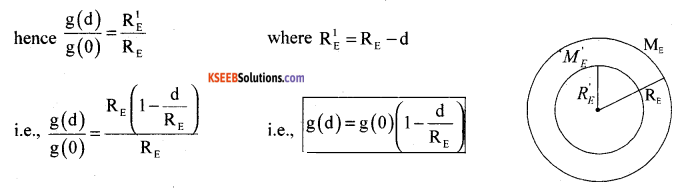Note : For d = RE (at the centre), $$\frac{\mathrm{d}}{\mathrm{R}_{\mathrm{E}}}$$ = 1 and g(RE) = 0.Question 31.
The energy omitted by a body on account of its temperature is called thermal radiation.

• They ate transverse wave in nature and travel in free space.
• They can be reflected and refracted.
• They do not heat intervening medium through which they pass.

Question 32.
Arrive at an expression for the time period of a simple pendulum.
The tangential component of force mg is mg sinθ.
Torque: -L(mgsinθ) = Iα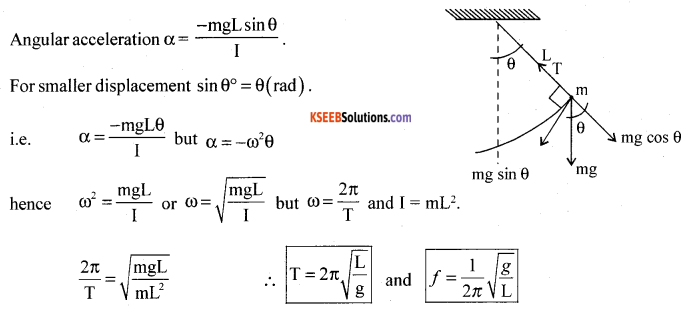VI. Answer any THREE of the following questions : ( 3 × 5 = 15 )

Question 33.
On a two – lane road, Car A is travelling with a speed of 36kmph. Two cars B and C approach car A in opposite directions with a speed of 54 kmph each. At a certain instant, when the distance AB is equal to AC, both being 1km, B decides to overtake A before C does. What minimum acceleration of car B is required to avoid an accident?
Speed of car A = 36 km/h = 36 × $$\frac{5}{18}$$ = 10 m/s
Speed of car B = speed of car C = 54 km / h = 54 × $$\frac{5}{18}$$ =15 m/s
Relative speed of car B with respect to car A is,
v = 15 – 10 = 5 m/s
relative speed of car C with respect to car A is
VCA = 15 + 10 = 25 m/s
AB = AC = 1 km = 1000m
w.k.t: s = ut
$$t=\frac{s}{u}=\frac{\mathrm{AC}}{\mathrm{V}_{\mathrm{AC}}}=\frac{1000}{25}=40 \mathrm{s}$$
Let ‘a’ be the acceleration of car ‘B’ for time t = 40s.
w.k.t
s = ut + $$\frac{1}{2}$$ at2
1000 = 5 × 40 + $$\frac{1}{2}$$ × a × 40 × 40
1000 = 200 +800a
∴ 800 a = 800
a = 1 ms-2Question 34.
A batsman deflects a ball by an angle of 45° without changing its initial speed which is equal to 54 kmph. What is the impulse imparted to the ball? If the time of contact . between the hall and the bat is 0.01s, what is the average force exerted by the bat on the ball? (Mass of the ball is 0.15 kg.)
Initial momentum of ball = mu
= $$\frac{0.15 \times 54 \times 1000}{3600}$$ = 0.15 × 15 = 2.25 kgms-1
Final momentum of ball = mu cos θ
= 2.25 × 0.707 ; cos 45° = 0.77 = 1.59 kg ms-1.
Impulse = 1.59 – 2.25 = – 0.66 kg ms-1
if Δt = 0.01s
average force F = $$\frac{\text { Impulse }}{\Delta t}=\frac{-0.66}{0.01}=-66 \mathrm{N}$$
F = -66N on the ball and + 66N on the bat

Question 35.
A rope of negligible mass is wound round a hollow cylinder of mass 3 kg and radius 40 cm. What is the angular acceleration of the cylinder if the rope is pulled with a force of 30 N? What is the linear acceleration of the rope? Assume that there is no slipping.
m = 3 kg
r = 40cm = 0.4m
F = 30N
The moment of inerties of the hallow cylinder about its geometer axis.
I = mr2
I = 3 × (0.4)2
I = 0.48 kg m2
(i) angular acceleration
w.k.t τ = Iα
But τ = 30 × 0.4
∴τ = 12Nm
∴ α = $$\frac{\tau}{\mathrm{I}}$$
α = $$\frac{12}{0.48}$$ = 25 rad s-1
(ii) liner acceleration
a = rα
a = 0.4 × 25
a = 10.0 ms-2

Question 36.
A steam engine delivers 5.4 × 108J of work per minute and services 3.6 × 109Jof heat per minute From its boiler. What is the efficiency .of the engine? How much heat is wasted per minute?
Work done by the steam engine per minute (W) = 5.4 × 108J Heat supplied from the boiler
H = 3.6 × 109J
Efficiency of the engine = $$\frac{\text { Output energy }}{\text { Input energy }}$$
∴η = $$\frac{W}{H}=\frac{5.4 \times 10^{8}}{3.6 \times 10^{9}}$$
∴η = 0.15
Hence the percentage efficiency of the engine is 15%
The amount of heat wasted = 3.6 × 109 – 5.4 × 108 = 36 × 108 – 5.4 × 108
= 30.6 × 108JQuestion 37.
A train, standing at the outer signal of a railway station blows a whistle of frequency 400 Hz in still air. (i) What is the frequency of the whistle for a platform observer when the train (a) approaches the platform with a speed of 10 ms-1, (b) recedes from the platform with a speed of 10 ms-1? (ii) What is the speed of sound in each case? The speed of sound in still air can be taken as 340 ms-1.
Given f=400 Hz, vw = 10 ms-1, v = 340 ms-1.
speed of sound = v + vw = 340 + 10 = 350 ms-1,
λ = $$\frac{v}{f}=\frac{340}{400}=0.85 \mathrm{m}$$
(i) Apparent frequency of sound f‘= $$\frac{\mathrm{fv}}{\left(\mathrm{v}+\mathrm{v}_{\mathrm{w}}\right)}$$
Here v0 =0 and vs = 0
i.e., f’ = $$\frac{400 \times 340}{350}$$ = 388.6 Hz
f’ ≈ 389 Hz
New wavelength of sound λ’
λ’ = $$\frac{v+w}{f}=\frac{350}{400}$$
i.e. λ’ = 0.875 m
Speed of sound as heard by an observer will be 350 ms-1 and
Δλ = 0.875 – 0.850 = 0.020 m.
(ii) When the air is still vw = 0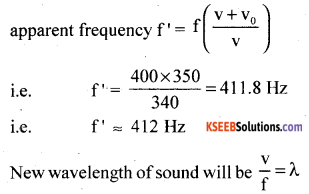i.e. λ’ = λ
There will be no change in the wavelength of sound w.r.t. the observer in motion. Relative velocity of sound w.r.t the observer = 350 ms-1.